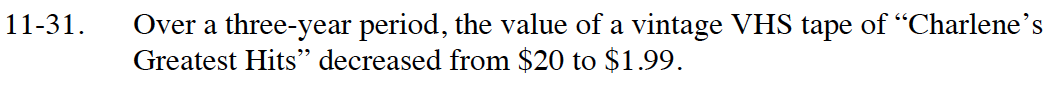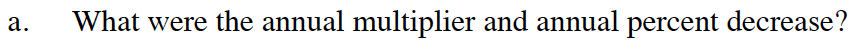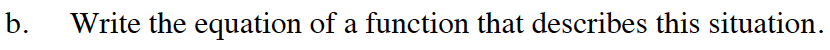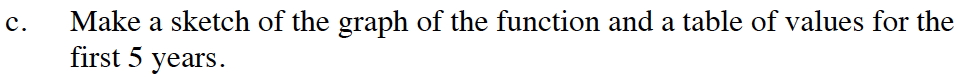Home > CC4 > Chapter 11 > Lesson 11.1.3 > Problem11-31

11-31.
1. Over a three-year period, the value of a vintage VHS tape of “Charlene’s Greatest Hits” decreased from $20 to$1.99. Homework Help ✎

1. What were the annual multiplier and annual percent decrease?

2. Write the equation of a function that describes this situation.

3. Make a sketch of the graph of the function and a table of values for the first 5 years.$20(m)(m)(m) =$1.99

The multiplier is 0.463.
How can you use that to find the percent decrease?Use the starting value and multiplier you found in part (a) to write the equation.

y = 20(0.463)x